## Fundamentals of Numerical Techniques for Static, Dynamic and Transient Analyses - Part 1# Fundamentals of Numerical Techniques for Static, Dynamic and Transient Analyses - Part 1

This, the first of two articles, compares the numerical aspects of dynamic and static solution types. The second article will discuss time varying (transient) problems and the pertinent features of implicit and explicit solutions.

## Statics

For a linear static analysis, the system equations can be represented as matrices of the form: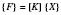The {F} matrix is 1 column wide (i.e. a vector) and is a numerical representation of the loads on the model. The [K] matrix is ‘square’; having as many rows as columns and, for a solid element model, its entries are the nodal stiffnesses in each direction. The {X} matrix is a single column vector of displacements and is the only unknown. To find it requires manipulation of the above equation, observing the rules of matrix algebra, giving: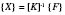Thus, to find the displacements within {X}, it is necessary to invert [K]; which accounts for the bulk of the processing required of the analysis. Once all the displacements are found, differentiation with respect to the different directions is required to obtain the strain matrix, which can be multiplied by a matrix of material properties to get the stresses.

## Eigenvalue Solutions: Modal Analyses

A static analysis is valid if the frequency of an applied load is significantly lower than the first natural frequency of the structure. If not, a vibration analysis is required, which can determine whether the structure is likely to resonate in response to the load.

Only modal analysis, which does not consider damping, is considered here. The aim of a modal analysis is to find the frequency values and displacement shapes of the natural frequencies of the structure. From the matrix equations given below, the eigenvalues are found, which are the squares of the natural frequencies; and the eigenvectors, which describe the maximum displaced shape the structure has when excited at this frequency. The eigenvectors cannot give actual values of displacement, only the relative displacements of each node in the model; hence the term mode shape. The displacement response of a structure to a specific forcing frequency would comprise some contribution from up to all the eigenvectors in varying proportions, dependant on the value of forcing frequency and the amount of damping for each mode.

For an undamped system the matrix equations are of the form: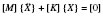The displacement vector {X} has been differentiated twice on the left hand side to produce the acceleration vector. The static problem considered before had only one solution for {X}; this problem has as many solutions as there are degrees of freedom: there are n solutions for {X}, where n is the number of degrees of freedom.

The solutions to the above equation are obtained by assuming the displacement vector is time dependent and has a simple harmonic form, thus: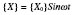So that: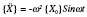The vector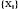is termed an eigenvector (of peak displacements over time) and represents the (mode) shape the item would assume if excited by a forcing frequency ofHz.

Substituting for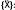: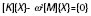or for the ith natural frequency, the solution can be written as: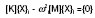Each one of these displacement solutions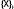, is a mode shape, which has a corresponding natural frequency.

A modal analysis will determine (within a small error due to the presence of damping) the proximity of any mode of interest to the frequency of the excitation force. Generally, the nearer the two, the more likely is resonance to occur and the more likely the chance of vibration induced failure. However, damping has a smearing effect and other mode shapes will also be evident to an extent. In general, modal analysis is used to check whether a resonant frequency is outside, or below a range of excitation loads.

Actual displacements can be obtained from a subsequent response analysis. This will thus provide a better assessment of the likelihood of failure from vibration, but will require damping values to be specified. These values are often difficult to obtain accurately.

## Buckling

Force equilibrium for a simple strut (the ‘Euler’ strut) gives a solution for displacements as a second order differential equation, the standard solution to which is trigonometric, implying multiple solution values. This illustrates that buckling can also be interpreted as an eigenvalue problem, which will be discussed in the second article.